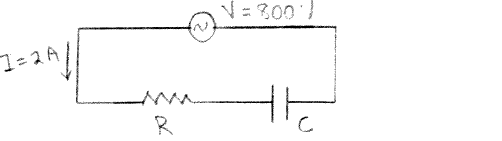# Problem: Consider the ac circuit shown in the sketch. R = 150 Ω and C = 5.0 x 10  -6 F. The source voltage amplitude is 800 V and the current amplitude is 2.0 A. What is the rate at which the source is supplying the electrical energy to the circuit?

84% (74 ratings)
###### Problem Details

Consider the ac circuit shown in the sketch. R = 150 Ω and C = 5.0 x 10  -6 F. The source voltage amplitude is 800 V and the current amplitude is 2.0 A.

What is the rate at which the source is supplying the electrical energy to the circuit?